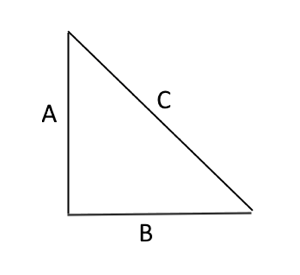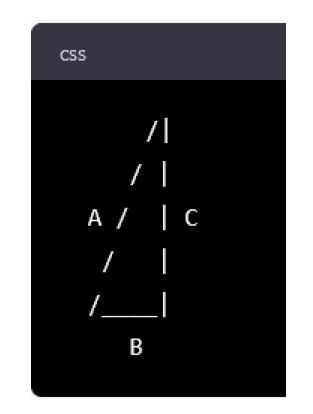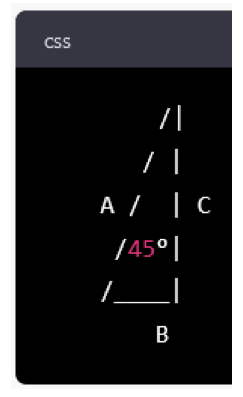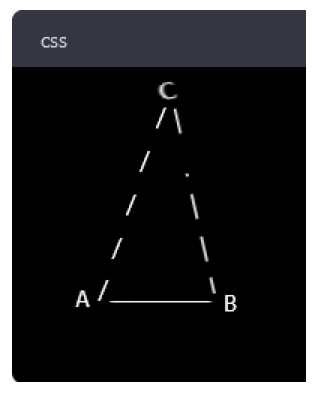Will the new update of an artificial intelligence platform be useful in teaching geometry?

On January 4, just after the holiday break, Education Next published my article “ChatGPT Is Not Ready to Teach Geometry (Yet).” I showed that version 3 of the popular chatbot developed by OpenAI (GPT-3) could give a passable explanation of Pythagoras’ theorem, but had serious limitations when asked to do other things that math teachers commonly do. In particular, GPT-3 could not describe student misconceptions or catch certain student errors. It couldn’t visualize a triangle or do anything equivalent, and it made a surprising computational error when squaring a number. I concluded that, despite the clear disruptive potential of AI for education, GPT-3 had little practical use for teaching geometry and might actually do more harm than good.

On March 14, OpenAI released version 4 of ChatGPT (GPT-4) to paid subscribers, and within days friends and colleagues—including EdNext’s managing editor—were telling me I ought to take a second look. “GPT-4 can do math now!” one friend told me. Indeed, OpenAI had published results claiming that GPT-4 could score above the 40th percentile on the AP Calculus BC exam and above the 80th percentile on the SAT Math exam, the AP Statistics exam and the AP Microeconomics exam. In addition, GPT-4 has the ability to combine graphics and text.

So it seemed worth asking again whether GPT-4 is ready to teach geometry.

The answer was still no. GPT4 started out all right, but within a few minutes it was suggesting that Pythagoras’ theorem wasn’t limited to right triangles. And by the end it was painted into a corner where it couldn’t give a straight answer about whether a triangle had a right angle or which side of a triangle was the longest.

But by the time I finished writing this article, there was a new announcement that could improve performance a lot. GPT will soon be able to connect to Wolfram Alpha, another program that’s much better at math.

Its Explanation is Fluent, but Not Entirely Accurate or Accessible

I started with the same question that I had asked GPT-3 over the holidays:

Explain how to use Pythagoras’ theorem in terms that a middle school math student would understand.

GPT-4 offered a longer and more cheerful explanation than GPT-3 had:

Pythagoras’ theorem is a math rule that tells us how to find the length of one side of a right triangle if we know the lengths of the other two sides.

So, what’s a right triangle? It’s a special kind of triangle that has one angle that measures exactly 90 degrees, like the corner of a square. You can tell if a triangle is a right triangle if one of its angles is a square corner.

Now, the theorem says that if you take the length of the shortest side of the right triangle (we call that side the “base”) and square it (multiply it by itself), and then take the length of the other side of the triangle (we call that side the “height”) and square it, and then add those two numbers together, you get the square of the length of the longest side of the triangle (which we call the “hypotenuse”).

It’s an OK explanation, but not every detail was correct. Contrary to GPT-4’s definition, the “base” is not necessarily the shortest side, and in fact it’s hard to see what the word “base” means unless you draw the triangle and orient it a certain way. One of GPT-4’s new features is the ability to integrate pictures and text, but it didn’t make use of that here. It would later.

GPT-4 summed up:

That might sound a little complicated, but it’s actually pretty simple. It means that if you know the lengths of two sides of a right triangle, you can figure out the length of the third side by using this formula. And that’s Pythagoras’ theorem!

What formula? GPT-4 hadn’t presented a formula in symbolic form, and although seeing a formula doesn’t always help students, it would have helped here. In fact, without a formula that can be rearranged to solve for different terms, it’s hard to see how knowing the length of any two sides would let you figure out the third.

Overall, although GPT-4 did a great job keeping its language at a middle school level, I doubt that a middle schooler unfamiliar with Pythagoras’ theorem would really get what GPT-4 was talking about.

Give an example.

And it did:

Sure, let’s say we have a right triangle with a base of 3 units and a height of 4 units. We want to find the length of the hypotenuse.

Using Pythagoras’ theorem, we can find the length of the hypotenuse as follows:

Square the length of the base: 3^2 = 9
Square the length of the height: 4^2 = 16
Add those two numbers together: 9 + 16 = 25
Take the square root of that sum: √25 = 5

So the length of the hypotenuse of this right triangle is 5 units.

The example is correct and detailed, and GPT-4 used bullet points to improve readability—something GPT-3 hadn’t done over the holiday. That said, it still didn’t state the formula or draw a picture, so a student might find the calculations a little mysterious if they’d never seen Pythagoras’ theorem before.

What if you just know the length of the hypotenuse? Can you use that to get the lengths of the legs?

GPT-4 began:

Yes…

But technically the correct answer is No. If you just know the hypotenuse, that is not enough to get the length of both legs. In addition to the hypotenuse, you need one more leg or angle (in addition to the right angle) to get the length of the final leg.

To be fair, the rest of GPT-4’s answer suggested that it knew this (to the extent that a chatbot “knows” anything). Perhaps GPT-4 was just being over-agreeable, like an upbeat teacher who doesn’t like to demoralize students by too often saying No. Here’s GPT-4’s full answer:

Yes, you can use Pythagoras’ theorem to find the lengths of the legs of a right triangle if you know the length of the hypotenuse and one of the legs.

Let’s say you have a right triangle with a hypotenuse of 10 units and one leg that measures 6 units. We can use Pythagoras’ theorem to find the length of the other leg as follows:

Square the length of the known leg: 6^2 = 36
Square the length of the hypotenuse: 10^2 = 100
Subtract the squared length of the known leg from the squared length of the hypotenuse: 100 – 36 = 64
Take the square root of that difference: √64 = 8

So the length of the unknown leg of this right triangle is 8 units.

Since all GPT-4’s examples so far involved variants of the classic 3-4-5 right triangle, I decided to give it an example with decimal numbers:

Suppose the hypotenuse of a right triangle is 7.1 miles long, and one of the legs is 3.85 miles long. How long is the other leg?

And after a few paragraphs of calculation GPT-4 gave the correct answer, 5.97—avoiding a computational error that had caused its predecessor, GPT-3, to give a slightly incorrect answer, 5.93, when I asked the same question over the holidays.

Its Discussion of Misconceptions Has Not Improved

As I wrote over the holidays, though, there’s more to teaching than giving correct explanations and examples. Teachers must also be able to field errors and misconceptions that might surface as students learn. Over the holidays, GPT-3 had bobbled when I asked this question:

What are some common misunderstandings of Pythagoras’ theorem?

GPT-4 gave a better answer when I asked this same question in March, but before I could copy it into my article, ChatGPT went down for a few days, and when it came back up my history was gone.

So I asked the question again, and now GPT-4 gave an answer that was just as bad as the one GPT-3 had given over the holidays. GPT-4  listed five “misconceptions,” but most of them were not misconceptions at all. To take things slightly out of order, GPT-4 best example of a misconception was this:

The theorem does not work for all triangle shapes.

That is correct, but then GPT-4 went slightly off the rails:

Another common misunderstanding is that Pythagoras’ theorem works for all types of right-angled triangles, regardless of their shape. However, the theorem only works for triangles where the sides form a 90-degree angle, and the side opposite the right angle is the longest side of the triangle.

“Wait,” I asked, “what other kind of right triangle is there?” GPT-4 answered:

I apologize for the confusion in my previous response. You are correct that the only type of right-angled triangle is one where one of the angles measures 90 degrees. Therefore, there is no other type of right triangle.

What I meant to convey was that Pythagoras’ theorem only applies to right-angled triangles, where one of the angles is a right angle (90 degrees), and where the side opposite the right angle is the longest side of the triangle.

Again, I apologize for any confusion my previous response may have caused. Thank you for bringing this to my attention.

This was a graceful recovery, but it amounted to an admission that the “misconception” GPT-4 had identified wasn’t a misconception at all. And it revealed that GPT-4 doesn’t have an entirely stable concept of what a right triangle is. In fact, it really doesn’t have a “concept” of triangles at all.

A debate currently raging is whether GPT-4’s ability to talk fluently about a wide variety of topics means that it really understands those topics in some way that gets behind the language. For example, OpenAI Chief Scientist Ilya Sutskever has asked: “What does it mean to predict the next token well enough? … It means that you understand the underlying reality that led to the creation of that token.”

In the case of right triangles, I think the answer is clearly that ChatGPT doesn’t understand them. Of course, it often doesn’t generate the right next token, either.

Here’s another “misconception” that GPT-4 highlighted:

The converse of Pythagoras’ theorem…(i.e., that a triangle with sides satisfying the equation of the theorem must be a right-angled triangle) is not always true. There are some triangles that satisfy the equation of the theorem but are not right-angled triangles.

“Really?” I asked. “Give an example of a non-right triangle that satisfies Pythagoras’ theorem.” And again GPT-4 backtracked:

I apologize for the error in my previous response. Pythagoras’ theorem only applies to right-angled triangles, and a non-right triangle cannot satisfy the theorem. I appreciate you catching that mistake.

GPT-4’s last two “misconceptions” were that Pythagoras’ theorem does not work with complex numbers or in non-Euclidean geometries. In fact, there are versions of Pythagoras’ theorem that work for complex numbers and some but not all non-Euclidean geometries. But I let that go because, clearly, the conversation had gone beyond a middle school level.

Randomness and Context Can Change ChatGPT’s Answers

Earlier I mentioned that ChatGPT went down for a couple of days and lost our history, and the fact that its answers changed when it came back up reveal a couple of things about how it works.

Second, GPT-4 depends on context. In my initial conversation with GPT-4, I asked it to keep things on a middle school level, and it did, but when it went down it lost that history, and when it came back up and I asked it for misconceptions, it started talking about complex numbers and non-Euclidean geometries—which are topics that rarely come up until college engineering or graduate math courses. I should have reestablished the context by asking it for a middle school explanation again. This is easy to forget.

It Still Falls Down When Provided Contradictory Information

Finally I tried to describe a right triangle without identifying it as such explicitly. The triangle I had in mind was this one:I began by saying:

Suppose a triangle has three sides called A, B, and C. A is 7 inches long and B is 7 inches long.

But then I made a mistake. I should have said:

The angle between A and C is 45 degrees, and so is the angle between B and C.

The angle between A and C is 45 degrees, and so is the angle between A and B.

What I described was not a right triangle, and in fact it’s not a triangle at all. There is no way to draw a triangle with sides and angles as I’ve described them. I made this same mistake when talking to GPT-3 over the holidays, and I decided to repeat it because GPT-3’s response had been so revealing. Any tutor or teacher has to field students’ errors, and there are several ways to do it. You can correct the error; you can explain or demonstrate why it’s an error; or you can ask students to repeat or correct themselves.

Neither GPT-3 nor GPT-4 did any of these things. Instead, they ran with the example and quickly got themselves into trouble.

After describing my impossible triangle, I asked:

What is the length of side C?

To solve this problem, we can use the law of cosines, which relates the sides and angles of a triangle.

And after some detailed calculations and emerged with the answer

The length of side C is approximately 3.63 inches.

“Great!” I said, a little disingenuously. “Can you draw the triangle?” GPT answered:

Sure! Here’s a rough sketch of the triangle:In this triangle, sides A and B are both 7 inches long, and the angle between them measures 45 degrees. Side C is the side opposite the 45 degree angle, and we have just calculated that its length is approximately 3.63 inches.

“I don’t understand,” I said. “If sides A and B are both 7 inches, why does A look so much longer than B? And why does C look longer than B if C is only 3.63 inches?”

Again GPT-3 apologized, but this time it couldn’t recover:

I apologize for any confusion caused by the sketch. My intention was to provide a rough drawing to help visualize the triangle, but I understand that the proportions may be difficult to interpret.

To clarify, in a right-angled triangle where two sides have equal length (as in this case with sides A and B), the hypotenuse (side C) will be longer than either of the other two sides. However, in this case…we found that it is approximately 3.63 inches. This means that it is shorter than either of the other two sides, A and B. Therefore, the proportions in the original sketch were not accurate.

I asked: “How can you say C is the longest side and then say that it is the shortest side? And how can you say C is the hypotenuse when A is the hypotenuse in the drawing?”

GPT-4 apologized.

Then I pointed out: “The angle between A and B doesn’t look like it’s 45 degrees.” GPT-4 apologized again and provided an “updated version” that looked exactly the same, except that the angle between A and B was labeled as 45 degrees when it clearly is not:I asked GPT-4 for the angle between A and C. After some calculations using the law of cosines, GPT-4 answered: 101 degrees. Then I asked for the angle between B and C. It answered 34 degrees, because the angles of a triangle must add to 180 degrees. Then I asked how this could be a right triangle if none of the angles was 90 degrees. I asked how the angle between A and C could be 101 degrees when it looks so much smaller than the other angles. I asked it how the angle between B and C could be 34 degrees when it looked for all the world like a right angle. And on and on.

GPT politely apologized, but it kept going in circles, drawing the same picture over and over and saying contradictory things about it. Interestingly, the picture was different than the picture GPT-4 had drawn before the service went down in March. That picture has disappeared from GPT-4’s history, but if my memory serves it looked something like this:It wasn’t a right triangle at all, and and GPT-4 couldn’t tell me clearly if the symbols A, B, and C represented the sides, the angles, or the vertices.

It may seem a little odd that GPT-4 would generate two such different pictures from the same prompt, but it makes more sense when you remember two things. First, every response from ChatGPT contains a random component. Second, no picture could be correct, since the information I supplied could not describe any real triangle.

Cause for Worry, Cause for Hope

When I first proposed to write about ChatGPT’s math ability in December, it may have been fair to respond that no one had proposed to use ChatGPT as a math tutor.

What a difference a few months have made. On Friday, Axios published a story titled “Sal Khan explains why GPT-4 is ready to be a tutor,” which reported that Newark, New Jersey, and Hobart, Indiana, had joined a pilot of a new product called Khanmigo, which uses ChatGPT to help tutor math. I don’t think ChatGPT is ready for this, but in the story Khan says that “it’s getting better,” and “stresses that Khanmigo didn’t just take GPT-4 out of the box — it also added its own ‘secret sauce’ to help avoid math errors.”

I hope it works. I hope kids in Newark and Hobart aren’t struggling with a chatbot that can be as confusing and confused as the one that I interacted with. I hope teachers in Newark and Hobart are keeping a close eye on the situation. I hope the districts have a well-defined and rigorous process for trying and evaluating new technologies that use “secret sauce.”

But I don’t know, and I’m a little worried.

Meanwhile, the technology continues to evolve. While the next version, GPT-4.5, is scheduled for release in September or October, what interests me more is the announcement of a plug-in that connects ChatGPT to Wolfram Alpha—an older technology, released in 2009, that solves math problems and helps answer questions involving math and data. This is exactly what I suggested in my January 4 article (not that anyone was looking to me for advice), and it sounds very promising because Wolfram Alpha is fundamentally built for math in a way that GPT, as a language model, is not.

The integration of ChatGPT and Wolfram Alpha hasn’t been released yet, but it may bring us closer to the “not-too-distant future” that I speculated about in January—a future when “we may have intelligent programs that can tutor students in specific subjects—programs that can converse in natural language [like ChatGPT], draw on deep and accurate representations of subjects like geometry [like Wolfram Alpha], and recognize and correct the common missteps and misconceptions that lead to wrong answers.”

At least I hope so. But we’ll have to see.

Paul von Hippel is a professor and associate dean of research in the LBJ School of Public Affairs at the University of Texas, Austin. This article is dedicated to his incomparable 10th-grade geometry teacher, Glenn Gabanski.

Last updated April 12, 2023